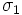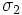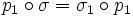# Isometry-invariant factorization

## Definition

### Symbol-free definition

An expression of a Riemannian manifold as a direct product of two Riemannian manifolds (up to isometry) is said to be isometry-invariant if the projections on both direct factors are isometry-invariant fibrations.

### Definition with symbols

Let$M = N \times P$ be Riemannian manifolds (the direct product is up to isometry). Let$p_1$ be the projection map from$M$ to$N$ and$p_2$ be the projection map from$M$ to$P$. Then we say that the factorization is isometry-invariant if for any isometry$\sigma$ of$M$, there are isometry$\sigma_1$ and$\sigma_2$ of$N$ and$P$ respectively, such that:$p_1 \circ \sigma = \sigma_1 \circ p_1$

and$p_2 \circ \sigma = \sigma_2 \circ p_2$